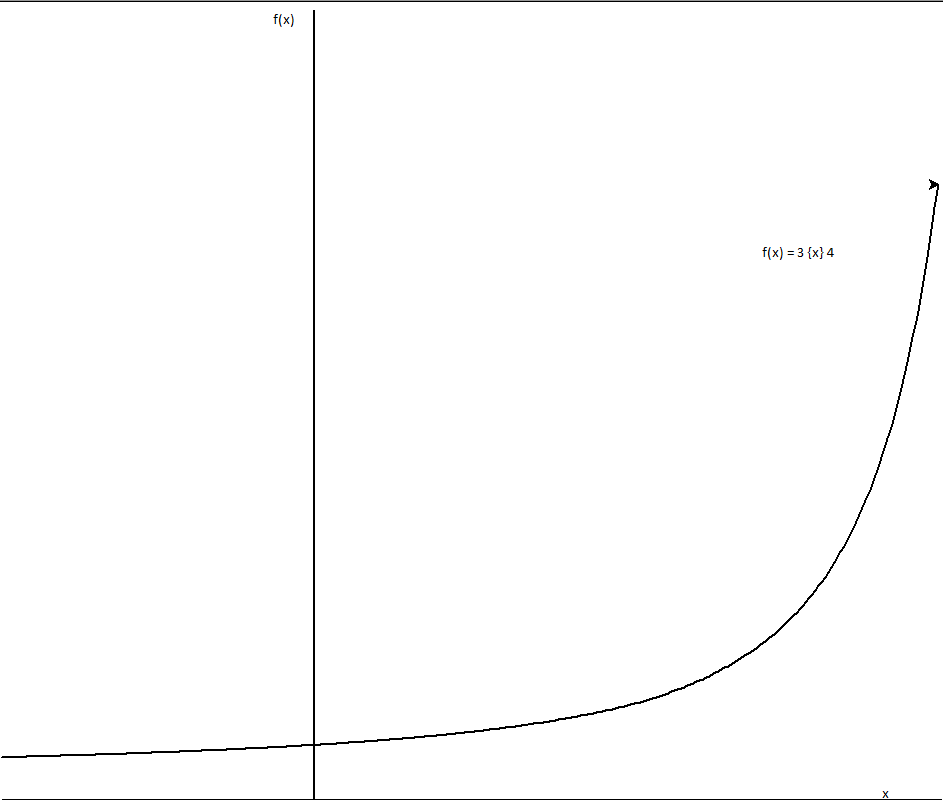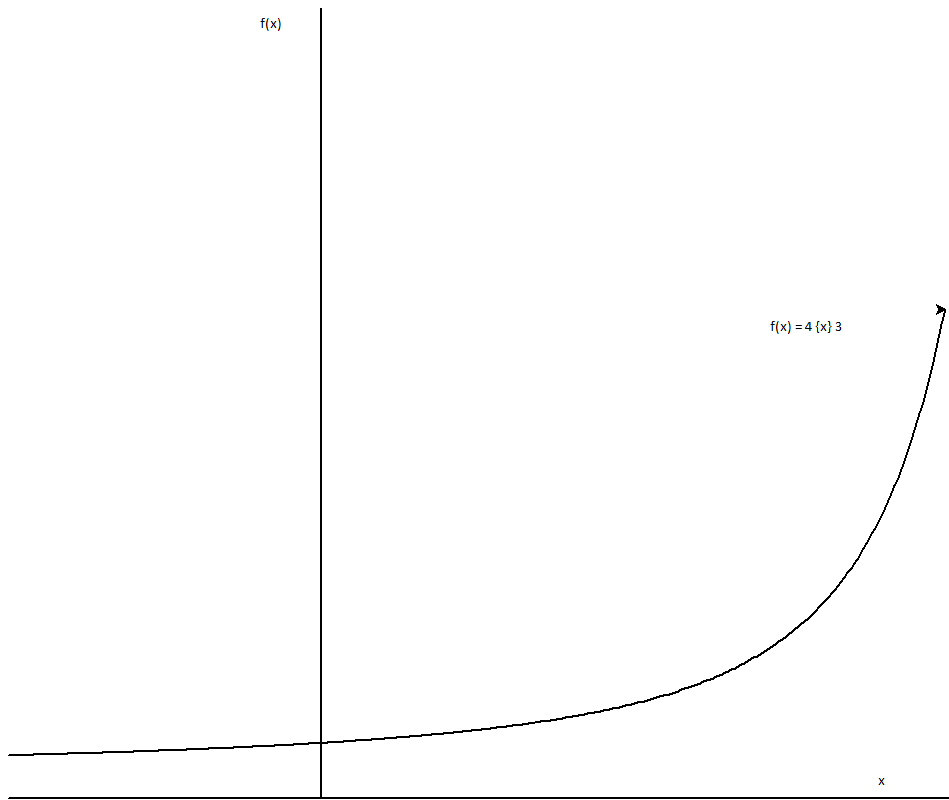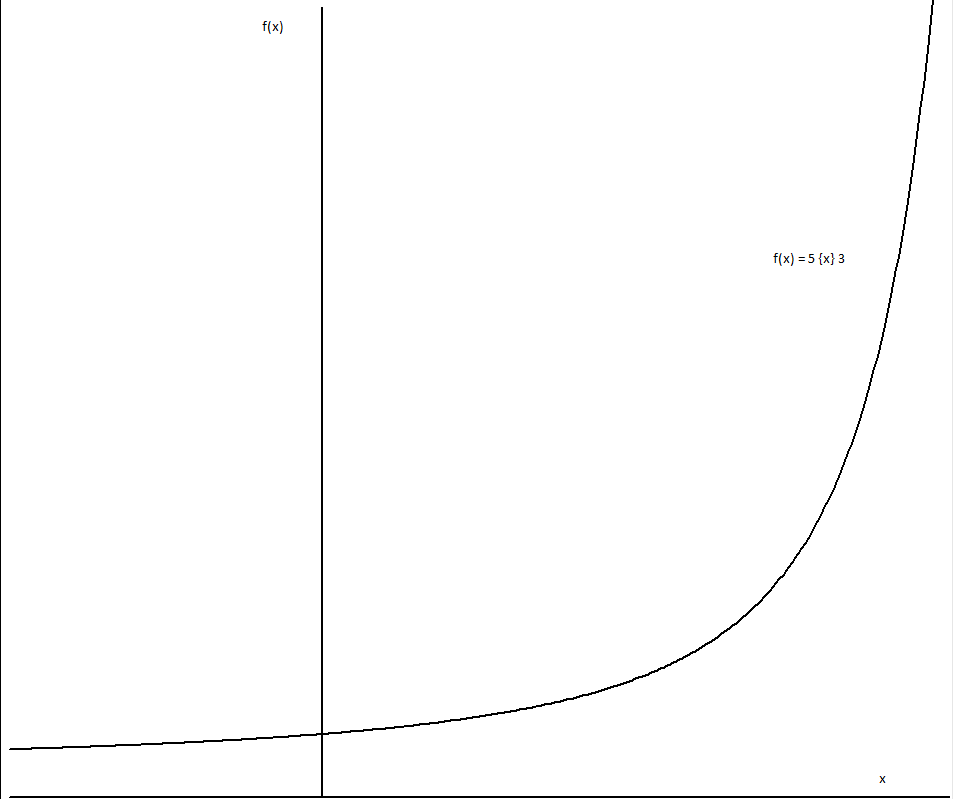Tetration Forum

Full Version: Rational operators (a {t} b); a,b > e solved
You're currently viewing a stripped down version of our content. View the full version with proper formatting.
Pages: 1 2 3 4
Well alas, logarithmic semi operators have paid off and have given a beautiful smooth curve over domain . This solution for rational operators is given by :

Which extends the ackerman function to domain real (given the restrictions provided).
the upper superfunction of is used (i.e: the cheta function).

Logarithmic semi-operators contain infinite rings and infinite abelian groups. In so far as {t} and {t-1} always form a ring and {t-1} is always an abelian group (therefore any operator greater than {1} is not commutative and is not abelian). There is an identity function S(t), however its values occur below e and are therefore still unknown for operators less than {1} (except at negative integers where it is a variant of infinity (therefore difficult to play with) and at 0 where it is 0). Greater than {1} operators have identity 1.

The difficulty is, if we use the lower superfunction of to define values less than e we get a hump in the middle of our transformation from . Therefore we have difficulty in defining an inverse for rational exponentiation. however, we still have a piecewise formula:

therefore rational roots, the inverse of rational exponentiation is defined so long as and .
rational division and rational subtraction is possible if and .

Here are some graphs, I'm sorry about their poor quality but I'm rather new to pari-gp so I don't know how to draw graphs using it. I'm stuck using python right now. Nonetheless here are the graphs.

the window for these ones is xmin = -1, xmax = 2, ymin = 0, ymax = 100If there's any transformation someone would like to see specifically, please just ask me. I wanted to do the transformation of as we slowly raise t, but the graph doesn't look too good since x > e.

Some numerical values:

(I know I'm not supposed to be able to calculate the second one, but that's the power of recursion)

I'm very excited by this, I wonder if anyone has any questions comments?

for more on rational operators in general, see the identities they follow on this thread http://math.eretrandre.org/tetrationforu...hp?tid=546

thanks, James

PS: thanks go to Sheldon for the taylor series approximations of cheta and its inverse which allowed for the calculations.
(06/06/2011, 02:45 AM)JmsNxn Wrote: [ -> ]Well alas, logarithmic semi operators have paid off and have given a beautiful smooth curve over domain . This solution for rational operators is given by :

Which extends the ackerman function to domain real (given the restrictions provided).
the upper superfunction of is used (i.e: the cheta function).
.....
Hey James,

Sounds very exciting. fyi, I admit I don't yet understand your new functions, but I made some graphs, and it looks very promising. I thought I'd post the following snippet of pari-gp code, which implements the rational operator function you posted, which can be used with mylatest kneser.gp code, which includes support. This would also make it easier for other to try your new function. If a<e, then I used sexpeta(invsexpeta(a)+t), instead of cheta(invcheta(a)+t). I think this is legal since the two are in many ways the same function when they are being to generate fractional iterations of eta. This expansive definition eliminates your restrictions, and covers all of the reals, working seamlessly from -infinity to infinity. It also matches your second example exactly.
Code:
expeta(t,a) = {
if (real(a)<exp(1), sexpeta(invsexpeta(a)+t), cheta(invcheta(a)+t));
}
fatb(a,t,b) = {
if (t<1,  return (expeta(t,expeta(-t,a)+expeta(-t,b))));
if (t>=1, return (expeta(t-1,b*expeta(1-t,a))));
}

I've gotten as far as quickly verifying that for t=0, we have addition, t=1, we have multiplication, and t=2 is exponentiation. The existing code has problems for invcheta(z) or invsexpeta(z), where z is too close to e, and invcheta(z)<=-1000, or invsexpeta(z)>1000. Other than that, it seems to work great. For example,
fatb(3,0,4)=7, which is 3+4
fatb(3,1,4)=12, which is 3x4
fatb(3,2,4)=81, which is 3^4
I wonder what it means that fatb(3,-1,4)=5.429897..?
Also, is there a smooth continuation to a function for t=3, which would be tetration?
- Sheldon
(06/06/2011, 04:39 AM)sheldonison Wrote: [ -> ]mylatest kneser.gp code, which includes support. This would also make it easier for other to try it.
Code:
fatb(a,t,b) = {
if (t>=1, return (cheta(invcheta(b*cheta(invcheta(a)+1-t))+t-1)));
if (t<1,  return (cheta(invcheta( cheta(invcheta(a)-t)+cheta(invcheta(b)-t) )+t)));
}

Oh that's great! I'd love to see what happens for complex operator values, I'll just modify your code slightly to say real(t) under the if conditions.

(06/06/2011, 04:39 AM)sheldonison Wrote: [ -> ]I've gotten as far as quickly verifying that for t=0, we have addition, t=1, we have multiplication, and t=2 is exponentiation.

for example,
fatb(3,0,4)=7, which is 3+4
fatb(3,1,4)=12, which is 3x4
fatb(3,2,4)=81, which is 3^4
what does it mean that fatb(3,-1,4)=5.429897..?
Is there a smooth continuation to a function for t=3, which would be tetration? if a<e=2.718, then it appears that the non integer nodes have complex values, though perhaps the sexpeta/invsexpeta functions could be used in place of cheta/invcheta (it might even be a smooth transition).
- Sheldon

fatb(3, -1, 4) = 5.429897, is the value of 3 delta 4; where deltation is the operator which satisfies the following conditions:
, it's commutative and addition is spread across it:
, and finally, it's defined by the following recursion formula:

Basically, the whole domain of operators from are designed to: continue the ring structure of {t-1} and {t} found across addition and multiplication, and multiplication and exponentiation; and are designed to provide a smooth extension of operators to the negative domain. It differs from zeration insofar as zeration is defined by proper recursion and it's discontinuous. It's better than zeration insofar as the series of operators can be continued infinitely.

As of now, it's possible to compute for a>e, and , which would be rational tetration. So actually yes, I can evaluate over domain
The formula is given by:

And lastly, I tried using sexp/slog base for a,b < e and the graph of is not smooth and there are two humps at about {0.5} and {1.5} which is very unflattering. I was surprised when the cheta function worked, but it did. I have to give myself time to think about it first, but right now I'm sure the extension for a,b <= e is right infront of our eyes.

Edit:
I edited your code and tested if
fatb(3, i, 3) = fatb(3, i+1, 2), and it does. Ackerman function is entering the complex plane me thinks.

Edit again:
Quote: if a<e=2.718, then it appears that the non integer nodes have complex values
I was wondering if you could explain what you mean by this? Is there a way to calculate a,b < e using the cheta function?
(06/06/2011, 05:34 AM)JmsNxn Wrote: [ -> ]....
Oh that's great! I'd love to see what happens for complex operator values, I'll just modify your code slightly to say real(t) under the if conditions.
Hey James, try my code snippet, which I updated while you were posting. It will work for values of a and b<e, seamlessly.
- Shel

(06/06/2011, 02:45 AM)JmsNxn Wrote: [ -> ]

But James, this is not analytic at , if we reformulate:

We can say:

where

is addition and composition of analytic functions, except this one function . The whole function can not be analytic. I wonder why it looks so smooth.

But then on the other hand there is a general problem with semioperators (note that your operator is 1 off the standard notation, i.e. {t}=[t+1]):
(a  0 = 1)
a  0 = a
a  0 = 0
a  0 = 1
a [n] 0 = 1 for n>3

or

(a  1 = 2)
a  1 = a+1
a  1 = a
a  1 = a for n>2

As soon as one defines a [t] 1 = a for t > 2, then the whole analytic function is already determined to be a, i.e. it must also be a for t=1 which is wrong.
Hence an analytic t |-> a[t]1 will not be constant but somehow meandering between the a's, which is somehow really strange.

But I see you gracefully avoided that problem by just defining it for a,b > ePS:
1. , This notation is ambiguous, compare . Please invent a better one!
2. , not \alpha but \circ belongs in the exponent: . This notation is derived from the symbol for function composition .

(06/06/2011, 05:34 AM)JmsNxn Wrote: [ -> ]Oh that's great! I'd love to see what happens for complex operator values, I'll just modify your code slightly to say real(t) under the if conditions....
at t=1, which corresponds to multiplication, and is the boundary between the two definitions, it appears continuous in the complex plane (which is quite amazing), but it also looks like the function may not converge there. I tried generating a taylor series for fatb(2,t,3) around t=1, with different samping radii, and different numbers of samping points, and it looks like the derivatives grow without bounds, even though the function is continuous in the complex plane. So far, it appears analytic around t=0.5, or t=1.5.
- Shel

(06/06/2011, 06:53 AM)bo198214 Wrote: [ -> ]But James, this is not analytic at , if we reformulate:

We can say:

where

is addition and composition of analytic functions, except this one function . The whole function can not be analytic. I wonder why it looks so smooth.

I like your definition better--it seems sleeker. I was sort of aware that there was no way I was gonna produce an analytic function over the whole complex domain, I'm happy with analytic in a few regions.
Quote:But I see you gracefully avoided that problem by just defining it for a,b > ewell hopefully I'll be having to tackle that problem soon.

Quote:PS:
1. , This notation is ambiguous, compare . Please invent a better one!

Alright, from henceforth I shall refer to logarithmic semi operators with the following notation:

And the inverse is given by:

therefore:
etc etc..

Quote:2. , not \alpha but \circ belongs in the exponent: . This notation is derived from the symbol for function composition .

I knew there was something off about my equations. lol

(06/06/2011, 06:02 AM)sheldonison Wrote: [ -> ]Hey James, try my code snippet, which I updated while you were posting. It will work for values of a and b<e, seamlessly.
- Shel

I'm wary about using for defining bases less than e. My complaints are explained by the following points:

fatb(e+0.0001, 2, pi*I) = -0.999999 - 0.00115551*I
fatb(e+0.0001, 1.8, pi*I) = -1.883265702 - 0.00194696*I
fatb(e+0.0001, 1.5, pi*I) = -5.707515375 - 0.011242371*I
fatb(e+0.0001, 1.3, pi*I) = -4.091499848 - 8.531525563*I
fatb(e+0.0001, 1.1, pi*I) = -1.002757644 - 8.536029475*I
fatb(e+0.0001, 1, pi*I) = -8.53659263001*I

Ignoring the drastic jumps in values, observe the hump that occurs in the real transformation. For no reason the values just spike to -5 randomly. This happens with all regular superfunctions of the logarithm. That's what makes the cheta function unique.

But I think, is it possible to create an upper superfunction for ?, perhaps it will give similar smooth results. Except it will be defined for a,b > 2... at least I think so.
(06/06/2011, 08:47 AM)JmsNxn Wrote: [ -> ]I was sort of aware that there was no way I was gonna produce an analytic function over the whole complex domain, I'm happy with analytic in a few regions.
Well not on the whole complex plane, but on the real axis, wouldnt that be nice?
I anyway wonder whether thats possible at all.
As is not even differentiable at 1, I wonder whether f is. Did you compare the derivations from left and right?

Quote:Alright, from henceforth I shall refer to logarithmic semi operators with the following notation:

oh I thought }t{ was kinda the inverse in t. But as I see that it is not ... there are already conventions that we use on the forum, even ASCII capable:
a  b = a+ b
a  b = a*b
a  b = a^b

so the operator is [t] (one off from your {t}). But then for the inverse functions
b [t] x = y
y /[t] x = b
b [t]\ y = x

for example
x / y = x - y
x \ y = -x + y

x / p is the p-th superroot (which is not equal to x  1/p)
b \ x is the superlogarithm to base b.

This is also in sync with the convention for quasigroups, i.e. groups with non-associative operation but with left- and right-inverse. There the left- and right inverse are written as / and \.

what is the idea or intention behind working with base eta ?
(06/06/2011, 04:39 AM)sheldonison Wrote: [ -> ]I wonder what it means that fatb(3,-1,4)=5.429897..?
no, it isn't correct, sorry.
fatb(2,-1,2) = 2º2=4 -> 2+2=4

fatb(3,-1,3) = 3º3=5 -> 3+2=5

fatb(4,-1,3) = 4º3=5 - it's correct. because that's here down:
aºb
if a>b then a+1
if b>a then b+1
if a=b then a+2

Pages: 1 2 3 4